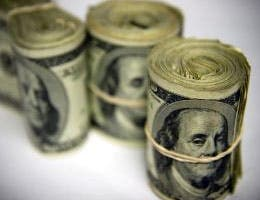# Frequent compounding boosts a CD’s APY

At Bankrate we strive to help you make smarter financial decisions. While we adhere to strict , this post may contain references to products from our partners. Here’s an explanation for

Dear Dr. Don,
I want to know the formula on how to get the daily compounded rate, the monthly compounded rate and the yearly compounded rate for a CD.

I have \$37,402 invested at 4 percent; they are paying me \$1,496 per year for a period of five years. The interest is given to me every year. I know this is simple interest, but I want to compare.
— Depositor Freed

Dear Depositor,
My editor gets a little nervous when I put formulas in columns. However, the formula for finding the annual percentage yield is pretty simple, so maybe he’ll let me do it just this once. Here’s the formula:

APY = (1 + i / m)m – 1

Where:
i is the annual (nominal) interest rate written in decimal form, and
m is the number of times interest compounds in a year.

APY daily = (1 + .04 / 365)365 – 1 = .04081 or 4.081 percent

APY monthly = (1 + .04 / 12)12 – 1 = .04074 or 4.074 percent

APY annually = (1 + .04 / 1)1 – 1 = .04 or 4 percent

Of course, there’s an easier way. Use Bankrate’s CD calculator and solve for the APY over a 12-month horizon based on the nominal interest rate and the appropriate compounding period. The calculator provides the same results as the formula above. As you can see from the calculations, the more frequent the compounding, the higher the APY. That’s because interest starts earning interest when it compounds in the account.

Appendix A in the Truth in Savings Act is more specific about how the annual percentage yield must be calculated when a financial institution advertises a yield on deposits. The idea behind APY in TISA is to present a yield that consumers can use to comparison shop among financial institutions. Bankrate also presents APYs when readers use its compare rates feature for CDs.

Want to see the big (financial) picture? Get Bankrate’s major financial stories of the week delivered to your inbox every Friday.

To ask a question of Dr. Don, go to the “Ask the Experts” page, and select one of these topics: “Financing a home,” “Saving & Investing” or “Money.” Read more Dr. Don columns for additional personal finance advice.